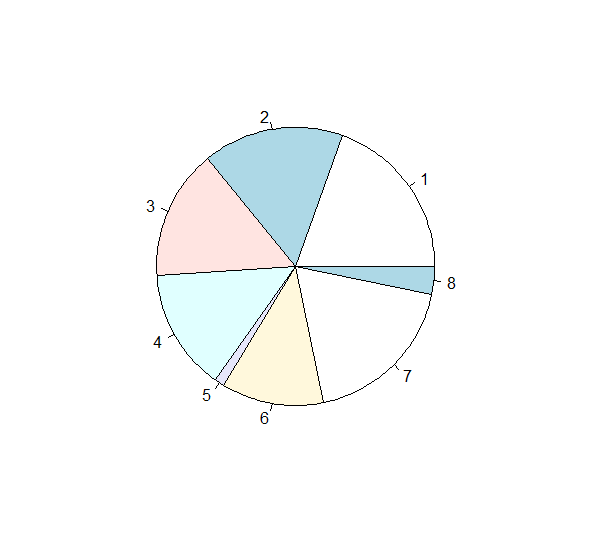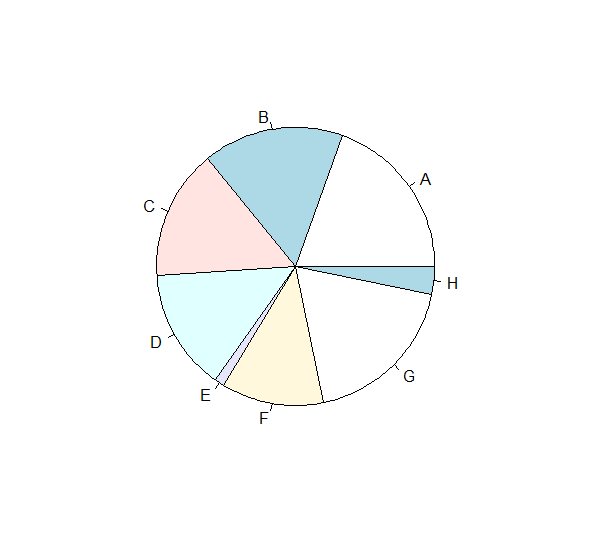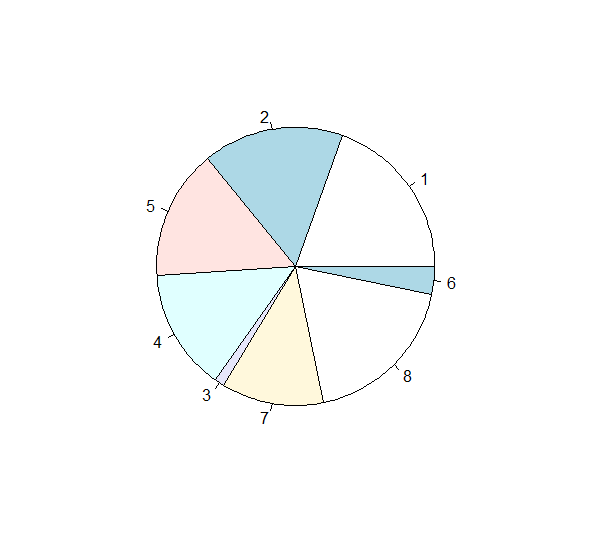# How to create pie chart in base R with labels?

A pie chart is a circular representation of data which is created for either nominal data or ordinal data. The slices in the pie chart depends on the magnitude of the data values. If we want to create a pie chart in base R with then pie function can be used along with labels argument.

Check out the Examples given below to understand how it can be done.

## Example

To create a pie chart in base R with labels, use the following command −

x<-sample(1:20,8)
pie(x)

## Output

If you execute the above given command, it generates the following Output −To create a pie chart in base R with labels add the following code to the above snippet −

x<-sample(1:20,8)
pie(x,labels=LETTERS[1:8])

## Output

If you execute all the above given snippets as a single program, it generates the following Output −To create a pie chart in base R with labels add the following code to the above snippet −

x<-sample(1:20,8)
pie(x,labels=c(1,2,5,4,3,7,8,6))

## Output

If you execute all the above given snippets as a single program, it generates the following Output −